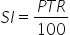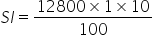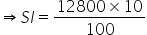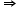Maths-
General
Easy

Question

# Ramesh invests  Rs12800 for three years at the rate of 10% per annum compound interest. Find (i) The sum due to Ramesh at the end of the 1st yearHint:

## The correct answer is: 14080 RUPEES

### Complete step by step solution:Let the principal amount P = 12800 RupeesHere we have R = 10% , T = 1 year P = 12800,  Rs and SI = ?(for 1st year, both simple interest and compound interest are the same)We calculate simple interest at the end of 1st year by the formula,…(i)where P is Principal amount, T is number of years and R is rate of interestOn substituting the known values in (i), we haveSI = 1280 RupeesSo interest for first year = Rs 1280Hence the sum of money at the end of first year = 12800 + 1280 = 14080 Rupees#### With Turito Foundation.#### Get an Expert Advice From Turito.